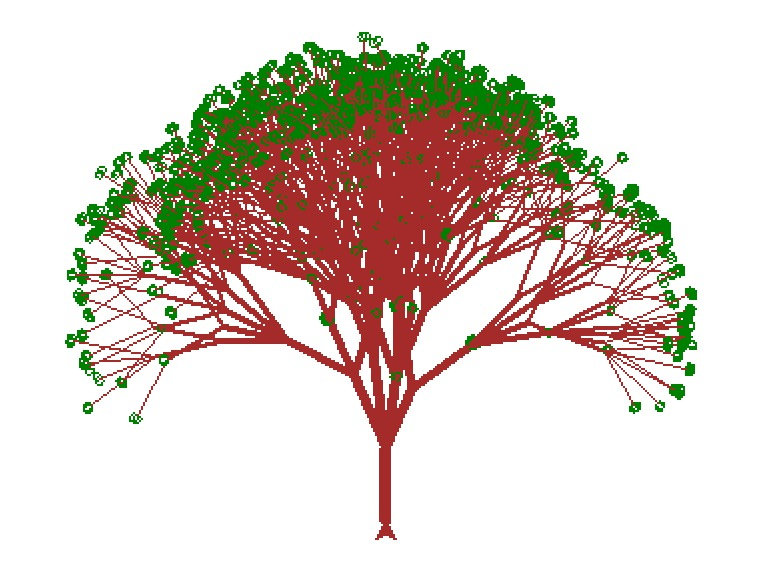# Draw treesFinal code
``````from turtle import *
from random import *

tracer(0, 0)
color("brown")
left(90)

minAngle    = 25
maxAngle    = 90
minLength   = 30
maxLength   = 45
minBranches = 2
maxBranches = 6

def tree(depth, branches, size, angle):
# Terminate at leaf or randomly
if depth < 1 or branches < 1 or random() < 0.1:
color("green")
right(90)
circle(2)
left(90)
color("brown")
return

# Set stroke thickness
width(depth)

# Draw root/trunk
forward(size)

# Turn to leftmost branch
left(0.5 * angle)
for i in range(branches):
# Turn to branch angle
if branches > 1:
right(i * angle / (branches - 1))

# Draw next depth tree
tree(depth - 1, randint(minBranches, maxBranches), randrange(minLength, maxLength), randrange(minAngle, maxAngle))

# Turn back to centre angle
if branches > 1:
left(i * angle / (branches - 1))

# Turn back to center
right(0.5 * angle)